# Group

Group of kids wanted to ride. When the children were divided into groups of 3 children 1 remain. When divided into groups of 4 children 1 remain. When divided into groups of 6 children 1 missed. After divided to groups of 5 children its OK. How many are there?

Result

n =  0

#### Solution:

Problem has no solutionLeave us a comment of example and its solution (i.e. if it is still somewhat unclear...):Be the first to comment!#### To solve this example are needed these knowledge from mathematics:

Do you want to calculate least common multiple two or more numbers? Do you solve Diofant problems and looking for a calculator of Diofant integer equations?

## Next similar examples:

1. Lesson exercising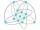The lesson of physical education, pupils are first divided into three groups so that each has the same number. The they redistributed, but into six groups. And again, it was the same number of children in each group. Finally they divided into nine equal gr
2. Exhibition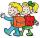The teacher paid for 280 Kč for 4.A students for admission to the exhibition. How many students were on the exhibition?
3. Foot in busIt was 102 people on the bus. 28 girls had two dogs. A 11 girls had one dog. At the next stop seceded 5 dogs (even with their owners). They got two boys together with three dogs. The bus drove one driver. How many foot were in bus?
4. SickSick Marcel already taken six tablets, which was a quarter of the total number of pills in the pack. How many pills were in the pack?
5. DoctorsIn the city operates 171 doctors. The city has 128934 citizens. How many citizens are per one doctor?
6. SymbolsIf 2*3 = 60 ; 3*4 = 120 and 4*5 = 200, what is 2*5?
7. The resultHow many times I decrease the number 1632 to get the result 24?
8. Math classificationIn 3A class are 27 students. One-third got a B in math and the rest got A. How many students received a B in math?
9. Perpetrator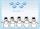The perpetrator is a number that is smaller than the number 80. It is a multiple of five and it is odd. If we added up the tens and ones of this number we get number 8.
10. Street numbersLada came to aunt. On the way he noticed that the houses on the left side of the street have odd numbers on the right side and even numbers. The street where he lives aunt, there are 5 houses with an even number, which contains at least one digit number 6.
11. Three Titanics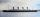Three steamers sailed from the same port on the same day. The first came back on the third day, fourth 4th day and the third returned sixth day. How many days after leaving the steamers met again in the harbor?
12. Athletes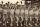Athletes standing in rows of eight. Ivan estimated that athletes are more than 120. Peter claimed that there are fewer than 130. Both are right. How many are athletes?
13. CagesHonza had three cages (black, silver, gold) and three animals (guinea pig, rat and puppy). There was one animal in each cage. The golden cage stood to the left of the black cage. The silver cage stood on the right of the guinea pig cage. The rat was in the
14. The largest numberFind the largest integer such that: 1. No figures is not repeat, 2. multiplication of every two digits is odd, 3. addition all digits is odd.
15. ProductResult of the product of the numbers 1, 2, 3, 1, 2, 0 is:Added together and write as decimal number: LXVII + MLXIV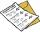The class has 22 students. 2 of which has assesement of 2. Count of assesement of 1 is three times more than count of students with assesement of 3. How many students has assesement of 1 and how many students has assesement of 1.